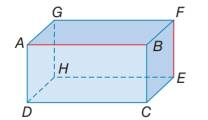Chapter 1.3, Problem 38E### Elementary Geometry for College St...

6th Edition
Daniel C. Alexander + 1 other
ISBN: 9781285195698

#### Solutions

Chapter
Section### Elementary Geometry for College St...

6th Edition
Daniel C. Alexander + 1 other
ISBN: 9781285195698
Textbook Problem
1 views

# A B ↔ and E F ↔ are said to be skew lines because they neither intersect nor are parallel. How many planes are determined bya) parallel lines A B and D C ?b) intersecting lines A B and B C ?c) skew lines A B and E F ?d) lines A B , B C , and D C ?e) points A, B, and F?f) points A, C, and H?g) points A, C, F, and H?Exercises 38 − 40

To determine

a)

To find:

Number of planes determined by the given information.

Explanation

Given:

The given figure is,

Approach:

Consider the given figure,

To determine

b)

To find:

Number of planes determined by the given information.

To determine

c)

To find:

Number of planes determined by the given information.

To determine

d)

To find:

Number of planes determined by the given information.

To determine

e)

To find:

Number of planes determined by the given information.

To determine

f)

To find:

Number of planes determined by the given information.

To determine

g)

To find:

Number of planes determined by the given information.

### Still sussing out bartleby?

Check out a sample textbook solution.

See a sample solution

#### The Solution to Your Study Problems

Bartleby provides explanations to thousands of textbook problems written by our experts, many with advanced degrees!

Get Started